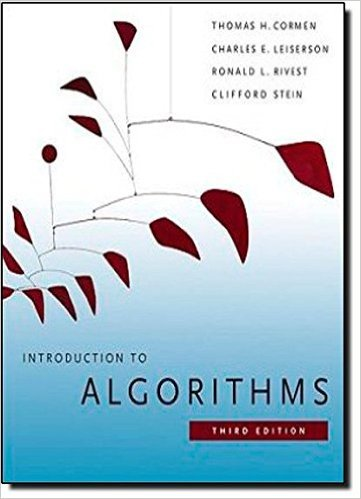×
Get Full Access to Introduction To Algorithms - 3 Edition - Chapter 2 - Problem 2-4
Get Full Access to Introduction To Algorithms - 3 Edition - Chapter 2 - Problem 2-4

×

# Inversions Let A1 : : n be an array of n distinct numbers.ISBN: 9780262033848 130

## Solution for problem 2-4 Chapter 2

Introduction to Algorithms | 3rd Edition

• Textbook Solutions
• 2901 Step-by-step solutions solved by professors and subject experts
• Get 24/7 help from StudySoup virtual teaching assistantsIntroduction to Algorithms | 3rd Edition

4 5 1 308 Reviews
13
4
Problem 2-4

Inversions Let A1 : : n be an array of n distinct numbers. If i Aj , then the pair .i; j / is called an inversion of A. a. List the five inversions of the array h2; 3; 8; 6; 1i. b. What array with elements from the set f1; 2; : : : ; ng has the most inversions? How many does it have? c. What is the relationship between the running time of insertion sort and the number of inversions in the input array? Justify your answer. d. Give an algorithm that determines the number of inversions in any permutation on n elements in .n lg n/ worst-case time. (Hint: Modify merge sort.)

Step-by-Step Solution:
Step 1 of 3

MTH 132 - Lecture 23 - Graphing How to use f’ and f’’ to graph 1. Determine domain 2. X intercept a. Solve for f(x) = 0 if possible 3. Y intercept a. Solve for f(0) 4. Critical points a. When derivative f’(x) = 0 5. Increasing or decreasing on the interval f(x) 6. Inflection points 7. Concavity f” 8. Vertical and horizontal asymptotes. 9. Use combined information to graph. Example: ● x − 3x 1. Domain: (-infinity, infinity) 2. X intercept: a. x(x^2 -3) = 0 √ √ b. X1 = 0, x2 = 3, x3 = − 3 3. Y intercept: a. f(0) = 0 4. Critical Points: a. 3x^2 - 3 b. 3(x^2-1) c. (x+1)(x-1) d. Cr

Step 2 of 3

Step 3 of 3

##### ISBN: 9780262033848

Unlock Textbook Solution